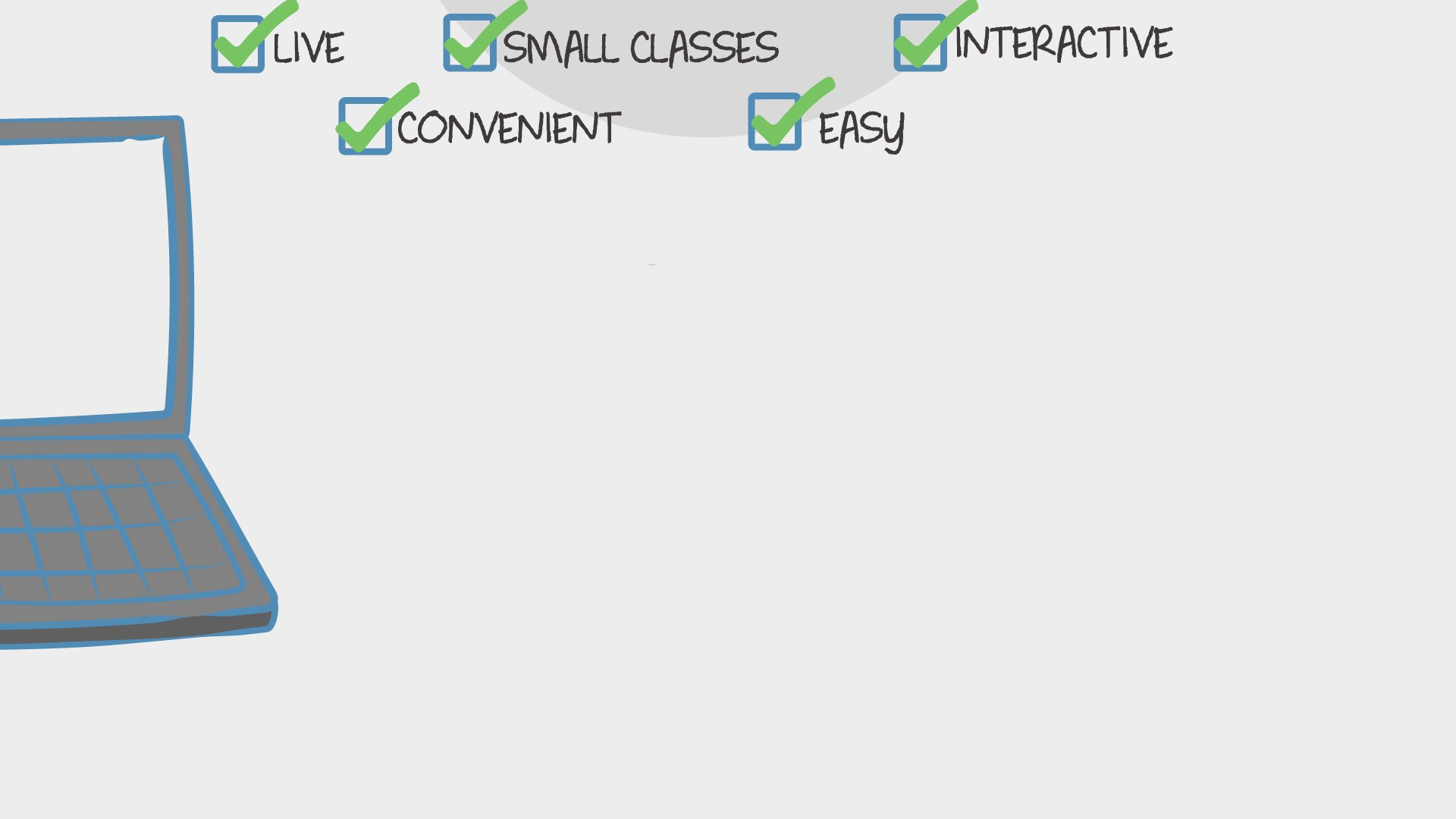Find Classes
Teach
Math

# Fifth Grade Math Skills Lessons With a Certified Teacher (2022-2023)

Students will learn fifth-grade math material in a supportive environment.
Sara Howell
67 total reviews for this teacher
4 reviews for this class
Completed by 15 learners25 minutes
per class
Twice per week
every week
9-12
year olds
1-5
learners per class
per learner - per class

### How does an "Ongoing" course work?

Meets on a weekly schedule, join any week, no need to catch up on previous material
Live video chats, recorded and monitored for safety and quality
Discussions via classroom forum and private messages with the teacher
Automatic payment every Sunday, cancel any time
Great for clubs and for practicing skillsPacific

### Available Times

Pacific
There are no open spots for this class, but you can request another time or scroll down to find more classes like this.

## Description

### Class Experience

```Students will be able to add and subtract decimals.
Students will be able to identify the properties of multiplication.
Students will be able to multiply numbers with zero at the end.
Students will be able to multiply multi-digit numbers.
Students will be able to multiply with decimals.
Students will be able to determine prime and composite numbers.
Students will be able to determine the factors of a number.
Students will be able to determine the greatest common factor.
Students will be able to divide numbers with zero at the end.
Students will be able to determine the divisor, dividend, and quotient.
Students will be able to divide numbers.
Students will be able to divide numbers with decimals.
Students will be able to divide division problems with two-digit divisors.
Students will be able to use orders of operations/PEMDAS.
Students will be able to determine the names of different types of angles.
Students will be able to measure angles.
Students will be able to determine equivalent fractions.
Students will be able to simplify fractions.
Students will be able to compare fractions.
Students will be able to determine the least common multiple.
Students will be able to determine the least common denominator.
Students will be able to convert mixed numbers to improper fractions.
Students will be able to convert improper fractions to mixed numbers.
Students will be able to add fractions with the same denominators.
Students will be able to add fractions with different denominators.
Students will be able to add mixed numbers.
Students will be able to subtract fractions with the same denominators.
Students will be able to subtract fractions with different denominators.
Students will be able to subtract mixed numbers.
Students will be able to multiply fractions with whole numbers and mixed numbers.
Students will be able to divide fractions with whole numbers and mixed numbers.
Students will be able to determine if a shape is closed or open.
Students will be able to identify quadrilaterals (parallelograms, rectangles, trapezoids, squares, and rhombuses).
Students will be able to determine the area of squares.
Students will be able to determine the area of rectangles.
Students will be able to determine the perimeter of squares.
Students will be able to determine the perimeter of rectangles.
Students will be able to solve area and perimeter word problems.
Students will be able to identify circles.
Students will be able to determine the circumference of a circle.
Students will be able to determine the diameter of a circle.
Students will be able to determine the radius of a circle.
Students will be able to identify the different types of triangles.
Students will be able to identify the area of triangles.
Students will be able to determine the surface area of rectangular prisms.
Students will be able to determine the surface area of cubes.
Students will be able to determine the volume of rectangular prisms.
Students will be able to determine the volume of cubes.
Students will be able to add, subtract, divide and multiply positive and negative integers.
Students will be able to identify equations and expressions.
Students will be able to solve equations using properties.
Students will be able to plot points on a coordinate plane.
Students will be able to identify the origin, x-axis, and x-axis on a coordinate plane.
Students will be able to convert inches, feet, yards, and miles.
Students will be able to determine when to use inches, feet, yards, and miles.
Students will be able to convert between centimeters, kilometers, and meters.
Students will be able to convert between cups, gallons, pints, and quarts.```
`I am a certified PK-6 general education teacher. I am also a certified PK-8 special education teacher. `
`I will occasionally provide extra worksheets for students if I feel they need additional help. I will always provide answers for students to look over their work. Any homework is optional. I create it after class so that it covers what the students need help with.`
`Students will need a whiteboard or paper to solve problems during class. `
`Learners will not need to use any apps or websites beyond the standard Outschool tools.`
`50 minutes per week in class, and maybe some time outside of class.`

## Teacher

Sara Howell
🇺🇸
Lives in the United States
Certified Teacher- B.S. in Early Childhood Education (PK-6) and Special Education (K-12)
67 total reviews
91 completed classes`Hello! I am Sara Howell. I live in a small town in Pennsylvania. I am a certified PK through sixth-grade general education teacher and PK- 12 grade special education teacher in both New Jersey and Pennsylvania. I graduated from Millersville... .css-1j9z6n7{position:relative;display:inline-block;font-family:'Ginto Normal',sans-serif;font-style:normal;font-weight:500;font-size:1.8rem;text-align:center;text-transform:none;height:auto;max-width:100%;white-space:nowrap;cursor:pointer;-webkit-user-select:none;-moz-user-select:none;-ms-user-select:none;user-select:none;outline:none;border:none;background:none;padding:0;-webkit-transition:all ease-in-out 0.05s,outline 0s,;transition:all ease-in-out 0.05s,outline 0s,;line-height:1;color:#380596;}.css-1j9z6n7:hover:not(:disabled),.css-1j9z6n7:focus:not(:disabled){color:#380596;-webkit-text-decoration:underline;text-decoration:underline;}.css-1j9z6n7:active:not(:disabled){color:#380596;}.css-1j9z6n7:disabled{color:#C2C2C2;cursor:default;}.css-1j9z6n7:focus-visible{outline-width:2px;outline-style:solid;outline-color:#4B01D4;outline-offset:2px;}`